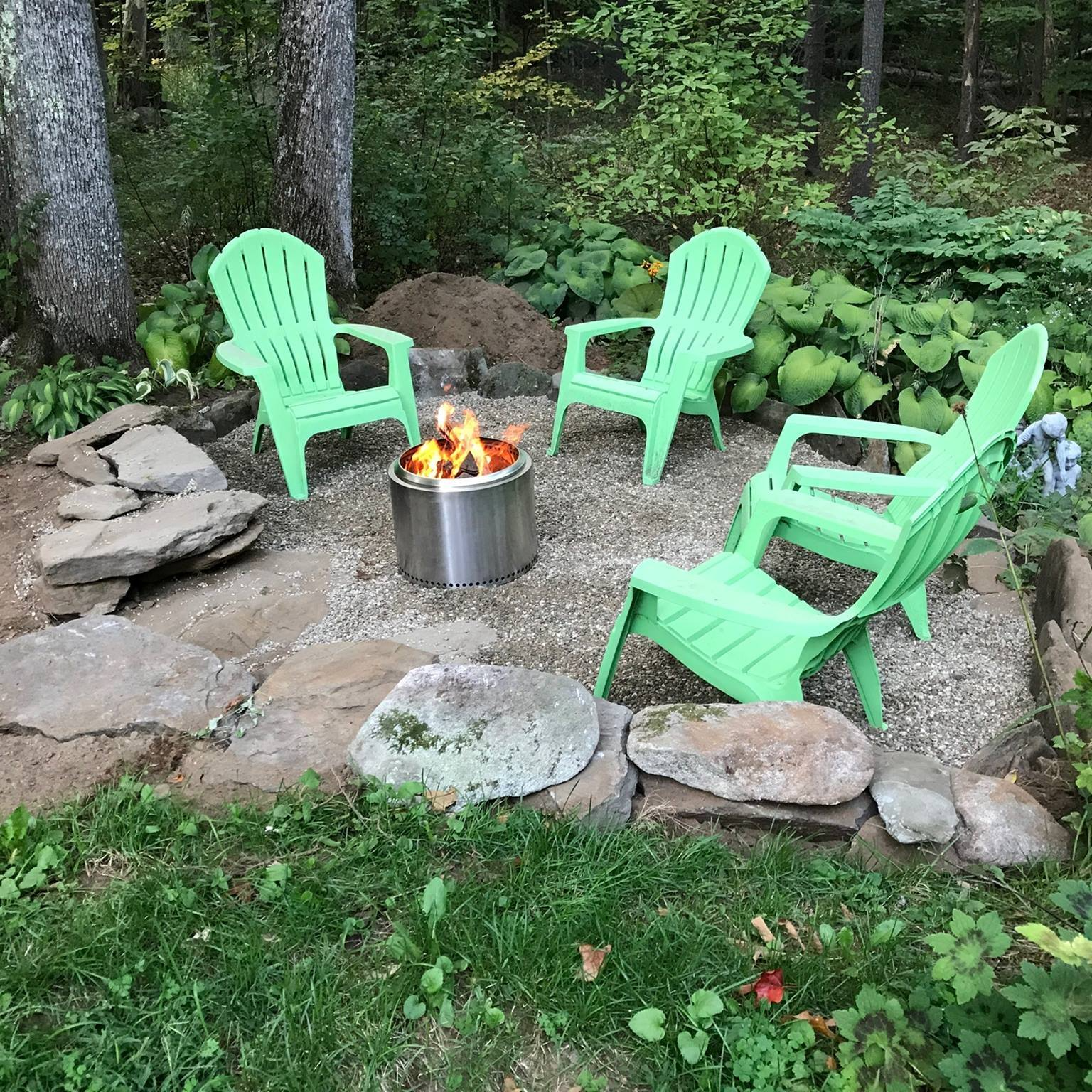# How to make plots with factor levels below the x-axis (bench-biology style)

The motivation for this post was to create a pipeline for generating publication-ready plots entirely within ggplot and avoid post-generation touch-ups in Illustrator or Inkscape. These scripts are a start. The ideal modification would be turning the chunks into functions with personalized detail so that a research team could quickly and efficiently generate multiple plots. I might try to turn the scripts into a very-general-but-not-ready-for-r-package function for my students. Continue to the whole post

# Paired line plots

load libraries make some fake data make a plot with ggplot ggplot scripts to draw figures like those in the Dynamic Ecology post Paired line plots (a.k.a. “reaction norms”) to visualize Likert data load libraries library(ggplot2) library(ggpubr) library(data.table) make some fake data set.seed(3) n <- 40 self <- rbinom(n, 5, 0.25) + 1 others <- self + rbinom(n, 3, 0.5) fd <- data.table(id=factor(rep(1:n, 2)), who=factor(rep(c("self", "others"), each=n)), stigma <- c(self, others)) make a plot with ggplot The students are identified by the column “id”.

# Interaction plots with ggplot2

ggpubr is a fantastic resource for teaching applied biostats because it makes ggplot a bit easier for students. I’m not super familiar with all that ggpubr can do, but I’m not sure it includes a good “interaction plot” function. Maybe I’m wrong. But if I’m not, here is a simple function to create a gg_interaction plot. The gg_interaction function returns a ggplot of the modeled means and standard errors and not the raw means and standard errors computed from each group independently.

# A simple ggplot of some measure against depth

set up The goal is to plot the measure of something, say O2 levels, against depth (soil or lake), with the measures taken on multiple days library(ggplot2) library(data.table) First – create fake data depths <- c(0, seq(10,100, by=10)) dates <- c("Jan-18", "Mar-18", "May-18", "Jul-18") x <- expand.grid(date=dates, depth=depths) n <- nrow(x) head(x) ## date depth ## 1 Jan-18 0 ## 2 Mar-18 0 ## 3 May-18 0 ## 4 Jul-18 0 ## 5 Jan-18 10 ## 6 Mar-18 10 X <- model.#### R doodles. Some ecology. Some physiology. Much fake data.

Thoughts on R, statistical best practices, and teaching applied statistics to Biology majors.

Jeff Walker, Professor of Biological Sciences

University of Southern Maine, Portland, Maine, United States